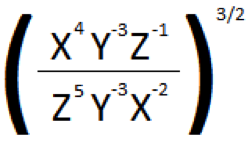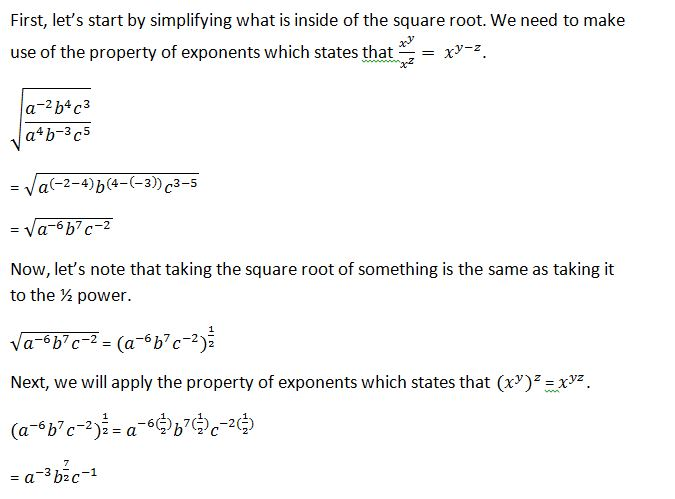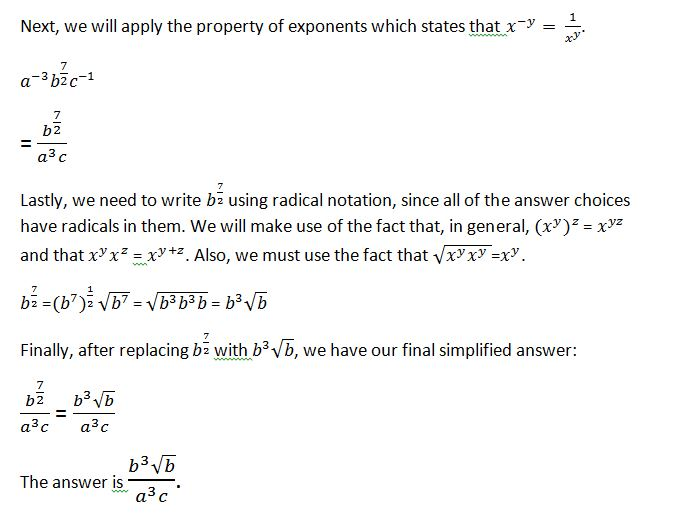## Example Questions

← Previous 1 3 4 5

### Example Question #1 : How To Divide Exponents

For all real numbers n, (2n * 2) / (2n * 2n) =

2n/2n

2n

21 – n

2n – 1

2

21 – n

Explanation:

(2n * 2) / (2n * 2n) simplifies to 2/2n or 21/2n.

When dividing exponents with the same base, you subtract the divisor from the dividend, giving 21–n.

### Example Question #2 : How To Divide Exponents

If x9/x3 = xn, solve for n.

9

7

12

6

3

6

Explanation:

When dividing terms with the same base, we can subtract the exponents:

9 – 3 = 6

### Example Question #3 : How To Divide Exponents

Simplify the following expression: (x2y4)/(x3y3z2)

xz2/y

xy/z2

z2xy

y/xz2

y/xz2

Explanation:

According to the rules of exponents, ax/ay  = ax-y

In this expression, we can follow this rule to simplify x2/xand y4/y3

x2–3 = x–1 = 1/x. y4–3 = y1 = y.

Therefore, y/xz2

### Example Question #4 : How To Divide Exponents

Simplify:x6y6z6

x/ z9

(z/x)/ 2

x/ z4

x3z/ y9

x/ z9

Explanation:

When dividing, subtract exponents (xa/xb = x(a – b).)  Therefore, the quantity in the parenthesis is: x(4 – (–2)) * y(–3 – (–3)) * z(–1 – 5) = x6/z6.  Raising  this to the 3/2 power results in multiplying the exponents by 3/2: x6 * 3/2/z6 * 3/2 = x9/z9.

### Example Question #1 : How To Divide Exponents

Half of the radioactive nuclei of a substance decays in a week.  If a sample started with 1010 nuclei, how many have decayed after 28 days?

1010

9.375 x 109

28 x 1010

106

6.25 x 108

9.375 x 109

Explanation:

If half of the sample decays each week: 1/2 is left after one week, 1/4 is left after two weeks, 1/8 is left after three weeks and 1/16 is left after four weeks (28 days.)  That means that 15/16 has decayed.  15/16 x 1010 = 9. 375 x 109

### Example Question #6 : How To Divide Exponents

1. 5. Simplify the problem (x4y2/x5)3

x/y

y6/x3

y5/x

x3y6

x4/y6

y6/x3

Explanation:

Properties of exponents suggests that when multiplying the same base, add the exponents, when dividing, subtract the exponents on bottom from those on top, and when raising an exponent to another power, multiply the exponents. Remember that (x4/x5) =  x–1 = 1/x; Still using order of operations (PEMDAS) we get the following:(x4y2/x5)3= (y2/x)3 = y6/(x3).

### Example Question #7 : How To Divide Exponents

If x7 / x-3/2 = xn, what is the value of n?

11/2

10/2

-21/2

21/2

17/2

17/2

Explanation:

x7 / x-3/2 = x7 (x+3/2) based on the fact that division changes the sign of an exponent.

x7 (x+3/2) =  x7+3/2 due to the additive property of exponent numbers that are multiplied.

7+3/2= 14/2 + 3/2 = 17/2 so

x7 / x-3/2 =  x7+3/2 = x17/2

Since x7 / x-3/2 = xn, xn = x17/2

So n = 17/2

### Example Question #8 : How To Divide Exponents

Simplify x2x4y/y2x

y/x5

x5/y3

7xy2

x5/y

x7y2

x5/y

Explanation:

1) According to the rules of exponents, one can add the exponents when adding to variables with the same base. So, x2x4 becomes x6.

2) The rules of exponents also state that if the bases are the same, one can substract the exponents when dividing. So, x6/x becomes x5. Similarly, y/ybecomes 1/y.

3) When combining these operations, one gets x5/y.

### Example Question #121 : Exponential Operations(b3√b)/(a3c)

(b3)/(a3c2)

a2b3√c

a3(b3√b)(c)

(b7)/(a3c)

(b3√b)/(a3c)

Explanation:### Example Question #10 : How To Divide Exponents

5/ 25 =

50

54 / 5

25

10

5